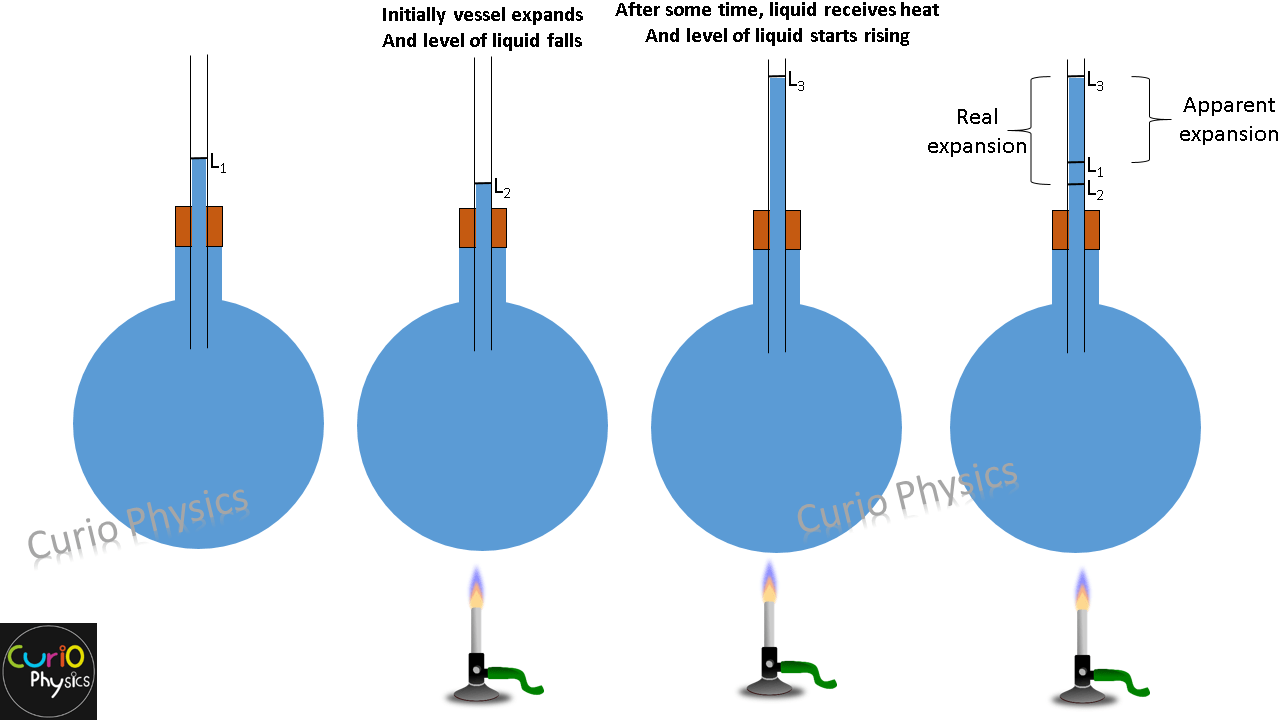# Thermal Expansion in liquids

Thermal expansion in liquids is similar to that of volume expansion in solids and is governed by governed by the similar relationship :-

V = V0( 1 + γΔT )

The bonds between separate molecules in liquids are usually less tight than solids, hence they expand more than solids for the same rise in temperature.

So γ for liquids is generally higher than that of solids.

Liquids are always heated along with a vessel which contains them so initially on heating the system (vessel + liquid), first the vessel gets heat and it expands due to which the level of liquid falls in the vessel. But after some time, the liquids also gets heated and expands more than vessel due to which the level of liquids starts rising.So liquids have two coefficients of volume expansion :-

1. Coefficient of apparent expansion γa :- Which is due to apparent expansion of liquid (expansion that appears to be, but is not), i.e., when expansion of vessel containing the liquid is not taken into account.
2. Coefficient of real expansion γr :- Which is due to the actual increase in volume of liquid, i.e., when expansion of vessel containing the liquid is taken into account.

Logically

ΔVreal = ΔVapparent + ΔVvessel

V0 ( γreal)ΔT = V0 apparent)ΔT + V0 vessel)ΔT

⇒  γreal = γapparent + γvessel

or

γr = γa + γv

As we have discussed above, that volume expansion coefficient of liquids γ, is generally higher (about 10 times) than that of solids and all the liquids generally increase in volume with increase in temperature. But water is an exception.The expansion of water is different from other liquids. From 0ºC to 4ºC water contracts (i.e., its volume decreases and density increases ⇒ γ is negative from 0ºC to 4ºC) and beyond 4ºC it expands (i.e., its volume increases and density decreases). Hence density of water is maximum (1000 kgm-3) at 4ºC.

This anomalous behavior of water causes ice to form first at the surface of a lake in cold weather. As winter approaches, the water temperature decreases initially at the surface. The water there sinks because of its increased density. Consequently, the surface reaches 0°C first and the lake becomes covered with ice. Aquatic life is able to survive the cold winter as the lake bottom remains unfrozen at a temperature of about 4°C.

Example. Coefficient of apparent expansion of a liquid is determined using 2 different vessels A & B and the values of coefficient of apparent expansion are γ1 and γ2 respectively. If the coefficient of linear expansion of vessel A is α then find coefficient of linear expansion of vessel B.

Solution. As we know that,  γr = γa + γv

For vessel A :-

γr = γ1 + γv

γr = γ1 + 3α     …..(1)

[because γ = 3α]

For vessel B :-

Let x be the coefficient of linear expansion of B, then

γr = γ2 + 3x     …..(2)

From equation (1) and (2), we get

γ1 + 3α = γ2 + 3x

⇒ x = (γ1 – γ2 + 3α)/3